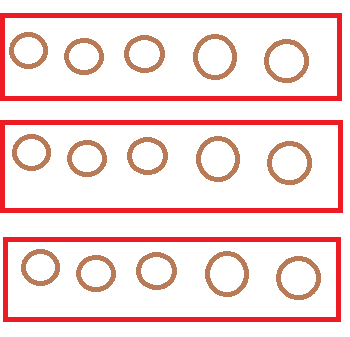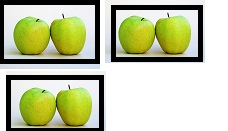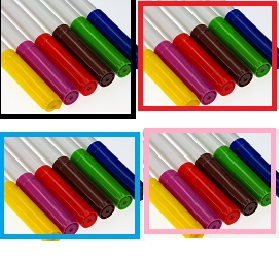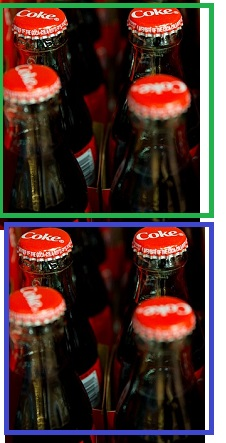# What is Multiplication? - Lesson for Kids

Instructor: Dina Albert
In this lesson we will unwrap the ~'magic~' of multiplication and apply what we know about adding to multiplying. We will look at times in real life when multiplication will come in handy!

## Math-Magician!

Before a magician can do magic tricks, he needs to learn the steps and tools to make the trick work. The same goes for a 'Math-Magician'! Multiplication is the trick we're learning today. We'll use addition in our steps and use some pictures as our tools. Let's become Math-Magicians!

Whether you knew it or not, sometimes you already do multiplication just by adding!

#### Example 1:

Take a look at these three groups of cookies:Since each group has 5 cookies in it, they are equal groups.

We could count each cookie one by one, but we can also add by 5's.

5 + 5 + 5 = 15 cookies total in the picture

#### Example 2:

You have three friends, and each of them wants two apples. You need to know how many apples to buy altogether. We can find the answer to this question by looking at the image of the apples.We can add by 2's to find the total number of apples. 2 + 2 + 2 = 6 apples total. So, you need to buy 6 apples.

#### Example 3: Buying Packs of MarkersIn the picture, each pack has 6 markers. You are buying 4 packs of them. Let's add by 6 to figure out how many markers you will have altogether.

6 + 6 + 6 + 6 = 24 total markers altogether

## Math Magic Time! Becoming a Math-Magician.

#### Multiplication is a different way to write how many groups we have in a picture and the amount of items in each group.

Let's look again at the picture of the apples:Earlier, we wrote 2 + 2 + 2

To rewrite this in multiplication terms:

1. Write down how many equal groups there are.

2. Write down the number of items in each group.

3. Put the symbol X in between the two numbers. This represents multiplying.

In the apple example, there are 3 (groups) X 2 (items in each group).

When we multiply 3 x 2, we are adding 3 groups of 2 apples together. In the picture, there are 6 apples total. So, 3 x 2 = 2 + 2 + 2 = 6 apples total

#### Let's look at a new example: Buying sodaFirst, we will count the number of equal groups. In this case, there are 2 equal groups.

Next, we will count the number of soda bottles in one group. There are 4 soda bottles.

To unlock this lesson you must be a Study.com Member.

### Register to view this lesson

Are you a student or a teacher?

#### See for yourself why 30 million people use Study.com

##### Become a Study.com member and start learning now.
Back
What teachers are saying about Study.com

### Earning College Credit

Did you know… We have over 200 college courses that prepare you to earn credit by exam that is accepted by over 1,500 colleges and universities. You can test out of the first two years of college and save thousands off your degree. Anyone can earn credit-by-exam regardless of age or education level.